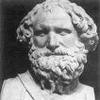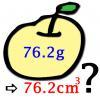#### You may also likeA 1 metre cube has one face on the ground and one face against a wall. A 4 metre ladder leans against the wall and just touches the cube. How high is the top of the ladder above the ground?### Archimedes and Numerical Roots

The problem is how did Archimedes calculate the lengths of the sides of the polygons which needed him to be able to calculate square roots?### More or Less?

Are these estimates of physical quantities accurate?

# Big and Small Numbers in Physics

##### Age 14 to 16Challenge Level

Physics makes use of numbers both small and large. Try these questions involving big and small numbers. You might need to use pieces of physical data not given in the question. Sometimes these questions involve estimation, so there will be no definitive 'correct' answer; on other occasions an exact answer will be appropriate. Use your judgement as seems appropriate in each context.

1. It is known that the value of $g$ on the moon is about one-sixth that on earth. How high do you think that you would be able to jump straight up on the surface of the moon?
2. The mass of an atom of lead is $3.44\times 10^{-22}\textrm{ g}$. Lead has a density of $11.35\textrm{ g cm}^{-3}$. How many atoms of lead are found in a single cubic centimetre of lead?
3. The earth orbits the sun on an almost circular path of average radius about $149\,598\,000\,000\textrm{ m}$. How fast is the earth moving relative to the sun?
4. The tallest buildings in the world are over $800\textrm{ m}$ high. If I dropped a cricket ball off the top of one of these, estimate how fast it would be moving when it hit the ground.
5. What weight of fuel would fit into a petrol tanker?
6. The charge on a proton is $1.6\times 10^{-19}\textrm{ C}$. What is the total sum of the positive charges in a litre of hydrochloric acid of $\mathrm{pH}$ $1.0$?
7. What is the mass of a molecule of water?
8. How many molecules of water are there in an ice cube?
9. Around $13.4$ billion years ago the universe became sufficiently cool that atoms formed and photons present at that time could propagate freely (this time was called the surface of last scattering). How far would one of these old photons have travelled by now?
10. How much energy is contained in the matter forming the earth?
NOTES AND BACKGROUND

An obvious part of the skill with applying mathematics to physics is to know the fundamental formulae and constants relevant to a problem. By not providing these pieces of information directly, you need to engage at a deeper level with the problems. You might not necessarily know all of the required formulae, but working out which parts you can and cannot do is all part of the problem solving process!